# Python Multiprocessing

## Python Programming Training Certification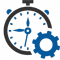Flexible Hours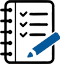100 Assignments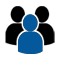Instructor Led online Training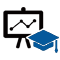50 LMS Access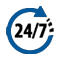24X7 Support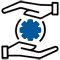100% Skill Level

### Enquire Now

4.9 out of 1000+ Ratings
Best Python Institute for Learning Python Course & Training, Live Project Training in Python with Django, Data Science and AI, Interview Assistance, Expert Coaching Trainers. Python Certification & Interview Assistance! Get free demo now!

### Course Overview

Python is one of the world’s top programming languages used today and Python training has become the most popular training across individuals. Training Basket’s Python Training & Certification course covers basic and advanced Python concepts and how to apply them in real-world applications.Python is a flexible and powerful open-source language that is easy to learn and consists of powerful libraries for data analysis and manipulation. Our Python training course content is curated by experts as per the standard Industry curriculum. The curriculum, coding challenges and real-life problems cover data operations in Python, strings, conditional statements, error handling, shell scripting, web scraping and the commonly used Python web framework Django. Take this Python training and certification course and become job-ready now.### Python Multiprocessing

In this article, we will learn how we can achieve multiprocessing using Python. We also discuss its advanced concepts.

What is Multiprocessing?

Multiprocessing is the ability of the system to run one or more processes in parallel. In simple words, multiprocessing uses the two or more CPU within the single computer system. This method is also capable to allocate the tasks between more than one process.

Processing units share the main memory and peripherals to process programs simultaneously. Multiprocessing Application breaks into smaller parts and runs independently. Each process is allocated to the processor by the operating system.

Python provides the built-in package called multiprocessing which supports swapping processes. Before working with the multiprocessing, we must aware with the process object.

Why Multiprocessing?

Multiprocessing is essential to perform the multiple tasks within the Computer system. Suppose a computer without multiprocessing or single processor. We assign various processes to that system at the same time.

It will then have to interrupt the previous task and move to another to keep all processes going. It is as simple as a chef is working alone in the kitchen. He has to do several tasks to cook food such as cutting, cleaning, cooking, kneading dough, baking, etc.

Therefore, multiprocessing is essential to perform several task at the same time without interruption. It also makes easy to track all the tasks. That is why the concept of multiprocessing is to arise.

• Multiprocessing can be represented as a computer with more than one central processor.
• A Multi-core processor refers to single computing component with two or more independent units.

In the multiprocessing, the CPU can assign multiple tasks at one each task has its own processor.

Multiprocessing In Python

Python provides the multiprocessing module to perform multiple tasks within the single system. It offers a user-friendly and intuitive API to work with the multiprocessing.

Let’s understand the simple example of multiple processing.

Example –

• from multiprocessing import Process
def disp():
print (‘Hello !! Welcome to Python Tutorial’)
if __name__ == ‘__main__’:
p = Process(target=disp)
p.start()
p.join()

Output:

• ‘Hello !! Welcome to Python Tutorial’

Explanation:

In the above code, we have imported the Process class then create the Process object within the disp() function. Then we started the process using the start() method and completed the process with the join() method. We can also pass the arguments in the declared function using the args keywords.

Let’s understand the following example of the multiprocessing with arguments.

Example – 2

• # Python multiprocessing example
# importing the multiprocessing module

import multiprocessing
def cube(n):
# This function will print the cube of the given number
print(“The Cube is: {}”.format(n * n * n))

def square(n):
# This function will print the square of the given number
print(“The Square is: {}”.format(n * n))

if __name__ == “__main__”:
# creating two processes
process1 = multiprocessing.Process(target= square, args=(5, ))
process2 = multiprocessing.Process(target= cube, args=(5, ))

# Here we start the process 1
process1.start()
# Here we start process 2

process2.start()

# The join() method is used to wait for process 1 to complete
process1.join()
# It is used to wait for process 1 to complete
process2.join()

# Print if both processes are completed
print(“Both processes are finished”)

Output:

• The Cube is: 125
The Square is: 25
Both processes are finished

Explanation –

In the above example, We created the two functions – the cube() function calculates the given number’s cube, and the square() function calculates the square of the given number.

Next, we defined the process object of the Process class that has two arguments. The first argument is a target that represents the function to be executed, and the second argument is args that represents the argument to be passed within the function.

• process1 = multiprocessing.Process(target= square, args=(5, ))
process2 = multiprocessing.Process(target= cube, args=(5, ))

We have used the start() method to start the process.

• process1.start()
process2.start()

As we can see in the output, it waits to completion of process one and then process 2. The last statement is executed after both processes are finished.

Python Multiprocessing Classes

Python multiprocessing module provides many classes which are commonly used for building parallel program. We will discuss its main classes – Process, Queue and Lock. We have already discussed the Process class in the previous example. Now we will discuss the Queue and Lock classes.

Let’s see the simple example of a get number of CPUs currently in the system.

Example –

• import multiprocessing
print(“The number of CPU currently working in system : “, multiprocessing.cpu_count())

Output:

• (‘The number of CPU currently woking in system : ‘, 32)

The above number of CPUs can vary for your pc. For us, the number of cores is 32.

Python Multiprocessing Using Queue Class

We know that Queue is important part of the data structure. Python multiprocessing is precisely the same as the data structure queue, which based on the “First-In-First-Out” concept. Queue generally stores the Python object and plays an essential role in sharing data between processes.

Queues are passed as a parameter in the Process’ target function to allow the process to consume data. The Queue provides the put() function to insert the data and get() function to get data from the queues. Let’s understand the following example.

Example –

• # Importing Queue Class

from multiprocessing import Queue

fruits = [‘Apple’, ‘Orange’, ‘Guava’, ‘Papaya’, ‘Banana’]
count = 1
# creating a queue object
queue = Queue()
print(‘pushing items to the queue:’)
for fr in fruits:
print(‘item no: ‘, count, ‘ ‘, fr)
queue.put(fr)
count += 1

print(‘\npopping items from the queue:’)
count = 0
while not queue.empty():
print(‘item no: ‘, count, ‘ ‘, queue.get())
count += 1

Output:

• pushing items to the queue:
(‘item no: ‘, 1, ‘ ‘, ‘Apple’)
(‘item no: ‘, 2, ‘ ‘, ‘Orange’)
(‘item no: ‘, 3, ‘ ‘, ‘Guava’)
(‘item no: ‘, 4, ‘ ‘, ‘Papaya’)
(‘item no: ‘, 5, ‘ ‘, ‘Banana’)

popping items from the queue:
(‘item no: ‘, 0, ‘ ‘, ‘Apple’)
(‘item no: ‘, 1, ‘ ‘, ‘Orange’)
(‘item no: ‘, 2, ‘ ‘, ‘Guava’)
(‘item no: ‘, 3, ‘ ‘, ‘Papaya’)
(‘item no: ‘, 4, ‘ ‘, ‘Banana’)

Explanation –

In the above code, we have imported the Queue class and initialized the list named fruits. Next, we assigned a count to 1. The count variable will count the total number of elements. Then, we created the queue object by calling the Queue() method. This object will used to perform operations in the Queue. In for loop, we inserted the elements one by one in the queue using the put() function and increased the count by 1 with each iteration of loop.

Python Multiprocessing Lock Class

The multiprocessing Lock class is used to acquire a lock on the process so that we can hold the other process to execute a similar code until the lock has been released. The Lock class performs mainly two tasks. The first is to acquire a lock using the acquire() function and the second is to release the lock using the release() function.

Python Multiprocessing Example

Suppose we have multiple tasks. So, we create two queues: the first queue will maintain the tasks, and the other will store the complete task log. The next step is to instantiate the processes to complete the task. As discussed previously, the Queue class is already synchronized, so we don’t need to acquire a lock using the Lock class.

In the following example, we will merge all the multiprocessing classes together. Let’s see the below example.

Example –

• from multiprocessing import Lock, Process, Queue, current_process
import time
import queue

while True:
try:

# The try block to catch task from the queue.
# The get_nowait() function is used to
# raise queue.Empty exception if the queue is empty.

except queue.Empty:

break
else:

# if no exception has been raised, the else block will execute

time.sleep(.5)
return True

def main():
total_number_of_processes = 3
number_of_processes = []

# defining number of processes
for w in range(total_number_of_processes):
number_of_processes.append(p)
p.start()

# completing process
for p in number_of_processes:
p.join()

# print the output

return True

if __name__ == ‘__main__’:
main()

Output:

Task no 0 is done by Process-1
Task no 1 is done by Process-3
Task no 2 is done by Process-2
Task no 3 is done by Process-1
Task no 4 is done by Process-3
Task no 5 is done by Process-2
Task no 6 is done by Process-1
Task no 7 is done by Process-3

Python Multiprocessing Pool

Python multiprocessing pool is essential for parallel execution of a function across multiple input values. It is also used to distribute the input data across processes (data parallelism). Consider the following example of a multiprocessing Pool.

Example –

• from multiprocessing import Pool
import time

w = ([“V”, 5], [“X”, 2], [“Y”, 1], [“Z”, 3])

def work_log(data_for_work):
print(” Process name is %s waiting time is %s seconds” % (data_for_work, data_for_work))
time.sleep(int(data_for_work))
print(” Process %s Executed.” % data_for_work)

def handler():
p = Pool(2)
p.map(work_log, w)

if __name__ == ‘__main__’:
handler()

Output:

• Process name is V waiting time is 5 seconds
Process V Executed.
Process name is X waiting time is 2 seconds
Process X Executed.
Process name is Y waiting time is 1 seconds
Process Y Executed.
Process name is Z waiting time is 3 seconds
Process Z Executed.

Let’s understand another example of the multiprocessing Pool.

Example – 2

• from multiprocessing import Pool
def fun(x):
return x*x

if __name__ == ‘__main__’:
with Pool(5) as p:
print(p.map(fun, [1, 2, 3]))

Output:

• [1, 8, 27]

Proxy Objects

The proxy objects are referred to as shared objects which reside in a different process. This object is also called as a proxy. Multiple proxy objects might have a similar referent. A proxy object consists of various methods which are used to invoked corresponding methods of its referent. Below is the example of proxy objects.

Example –

• from multiprocessing import Manager
manager = Manager()
l = manager.list([i*i for i in range(10)])
print(l)
print(repr(l))
print(l)
print(l[2:5])

Output:

• [0, 1, 4, 9, 16, 25, 36, 49, 64, 81]
<ListProxy object, typeid ‘list’ at 0x7f063621ea10>
16
[4, 9, 16]

The proxy objects are picklable so we can pass them between processes. These objects are also used for level of control over the synchronization.

Commonly Used Functions of Multiprocessing

So far, we have discussed the basic concepts of multiprocessing using Python. Multiprocessing is a broad topic itself and essential for performing various tasks within a single system. We are defining a few essential functions that are commonly used to achieve multiprocessing.

Method Description
pipe() The pipe() function returns a pair of connection objects.
run() The run() method is used to represent the process activities.
start() The start()method is used to start the process.
join([timeout]) The join() method is used to block the process until the process whose join() method is called terminates. The timeout is optional argument.
is_alive() It returns if process is alive.
terminate() As the name suggests, it is used to terminate the process. Always remember – the terminate() method is used in Linux, for Windows, we use TerminateProcess() method.
kill() This method is similar to the terminate() but using the SIGKILL signal on Unix.
close() This method is used to close the Process object and releases all resources associated with it.
qsize() It returns the approximate size of the queue.
empty() If queue is empty, it returns True.
full() It returns True, if queue is full.
get_await() This method is equivalent get(False).
get() This method is used to get elements from the queue. It removes and returns an element from queue.
put() This method is used to insert an element into the queue.
cpu_count() It returns the number of working CPU within the system.
current_process() It returns the Process object corresponding to the current process.
parent_process() It returns the parent Process object corresponding to the current process.
task_done() This function is used indicate that an enqueued task is completed.

### Recently Trained Students#### Jessica Biel

– Infosys

My instructor had sound Knowledge and used to puts a lot of effort that made the course as simple and easy as possible. I was aiming for with the help of the ZebLearn Online training imparted to me by this organization.#### Richard Harris

– ITC

I got my training from Zeblearn in the Python Certification Training, I would like to say that say he is one of the best trainers. He has not even trained me but also motivated me to explore more and the way he executed the project, in the end, was mind-blowing.

### Candidate’s Journey During Our Training Program#### Expert’s Advice & Selection of Module

Choosing the right type of module for the training is half the battle & Our Team of experts will help & guide you.#### Get Trained

Get Trained & Learn End to End Implementation from our Expert Trainer who are working on the same domain.#### Work on Projects

We Do make our student’s work on multiple case studies , scenario based tasks & projects in order to provide real-time exposure to them.#### Placements

We have a dedicated placement cell in order to provide placement assistance & relevant interviews to our candididates till selection